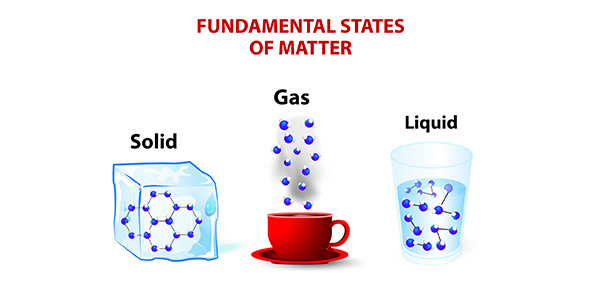# Matter And How It Changes - Unit B, Chapter 1

19 Questions | Total Attempts: 69SettingsThis is a quiz about matter and how it changes.

Related Topics
• 1.
In what way are all objects alike?
• A.

They take up space.

• B.

They have weight.

• C.

Both a and b.

• 2.
How can you describe matter?
• A.

You can describe them according to their characteristics such as color, size, or shape.

• B.

You can describe colors only.

• C.

You can describe size only.

• D.

You can describe shape only.

• 3.
How can you measure the properties of matter?
• A.

You can measure mass and volume using speakers on a stereo.

• B.

You can measure mass and volume using a ruler/meter stick, measuring cup, or balance.

• C.

You can measure mass and volume using a scale.

• 4.
What are some types of physical change?
• A.

Rusting and oxydation.

• B.

Burning and rusting.

• C.

A change in size, shape, or form.

• 5.
What are the three states of matter?
• A.

Solid

• B.

Liquid

• C.

Gas

• D.

All of the above

• 6.
How can matter change from one state to another?
• A.

Through a physical change such as melting ice to get liquid water.

• B.

Through a chemical change such as melting ice to get liquid water.

• C.

None of the above.

• 7.
How can one kind of matter change into another kind of matter?
• A.

When a physical change takes place.

• B.

When a chemical change takes place.

• C.

When a kinetic change takes place.

• 8.
How are chemical changes useful?
• A.

They are used to create plastics, clothing materials, and medicines.

• B.

• C.

They are used to create popsicles.

• 9.
All objects are made of _______________.
• A.

Mass

• B.

Matter

• C.

Volume

• 10.
Fur, bones, claws, and teeth are all kinds of matter that make up ________________.
• A.

Birds

• B.

Reptiles

• C.

Bears

• 11.
All matter takes up space and has _______________.
• A.

Air

• B.

Weight

• C.

Volume

• 12.
Red, hard, and round are all properties of __________.
• A.

Oranges

• B.

Baseballs

• C.

Apples

• 13.
The mass of an object is usually measured in ____________.
• A.

Volume

• B.

Hertz

• C.

Grams

• 14.
A tool for measuring mass is a __________________.
• A.

Thermometer

• B.

Gauge

• C.

Balance

• 15.
Length can be measured by a ______________.
• A.

Yardstick

• B.

Ruler

• C.

Yardstick or ruler

• 16.
Physical changes in matter involve _____________.
• A.

Shape

• B.

Color

• C.

Size

• D.

All of the above

• 17.
When liquid turns into a gas, it _________.
• A.

Evaporates

• B.

Condenses

• C.

Precipitates

• 18.
Melting ice does not involve a ___________.
• A.

Physical change

• B.

Chemical change

• C.

Kinetic change

• 19.
Which of your senses can you use to determine some properties of a strawberry?
• A.

Sight

• B.

Touch

• C.

Smell

• D.

All of the above